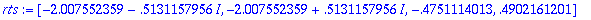Advice: Real values for a RootOf

Maple often returns solutions to various types of equations in terms of RootOf a polynomial. The allvalues function can be used to replace the RootOf with the roots of the polynomial. This will use symbolic solutions if that is possible (e.g. if the polynomial is of degree 4 or less), otherwise it uses floating-point approximations. In many cases this may be inconvenient because the symbolic solutions are very complicated. Moreover, it includes complex solutions, and you may wish to include only real solutions. An alternative, which always returns floating-point results for real solutions, is to use fsolve as follows.

Examples:

> solve({ y^2+2*y+x=0, y = x^2 - 1 });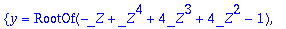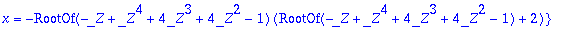Save this in a variable:

> q:= ":

Isolate the RootOf :

> ro:= op(indets(q,RootOf));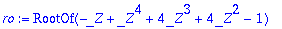Find a list of the real roots:

> rts:= [ fsolve(op(ro)) ];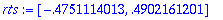Substitute into the solutions:

> map(t -> subs(ro=t,q), rts);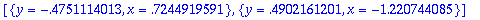You could obtain complex solutions in the same way, using fsolve with the complex option:

> rts:= [ fsolve(op(ro), _Z, complex) ];